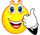## Wednesday, October 2, 2013

### Variables are not taken in a stringA query embedded with a unix variable is stored in the database table. When it is pulled out, it has a variable in it and it needs to be replaced by the variable passed to the script below.

Code:
1. select cast(cmd_string as varchar(1000)) from mytable where tabname='mytable'
Output from the query:
select char(substr(date(substr(MTH_BEG_DY_ID,1,4)||'-'||substr(MTH_BEG_DY_ID,5,2)||'-'||substr(MTH_BEG_DY_ID,7,2)) + 1 month,7,4)||substr(date(substr(MTH_BEG_DY_ID,1,4)| |'-'||substr(MTH_BEG_DY_ID,5,2)||'-'||substr(MTH_BEG_DY_ID,7,2)) + 1 month,1,2),6) from ECD.DT_MTH_DIM where MTH_DIM_ID=\$lowvalue with ur
2. Now this query is passed to a variable
lowsql="\${abovequery}"
echo lowsql:
select char(substr(date(substr(MTH_BEG_DY_ID,1,4)||'-'||substr(MTH_BEG_DY_ID,5,2)||'-'||substr(MTH_BEG_DY_ID,7,2)) + 1 month,7,4)||substr(date(substr(MTH_BEG_DY_ID,1,4)| |'-'||substr(MTH_BEG_DY_ID,5,2)||'-'||substr(MTH_BEG_DY_ID,7,2)) + 1 month,1,2),6) from ECD.DT_MTH_DIM where MTH_DIM_ID=\$lowvalue with ur
3. Now trying to replace \$lowvalue with the actual value:
function runq {
lowvalue=\$1
echo lowvalue \$lowvalue before
echo "lowsql before \$lowsql"
p=`echo "\$lowsql"`
echo p: \$p

runq 201302

4. Output from this function:
./makeq.ksh 201302
lowvalue 201302 before
lowsql before select char(substr(date(substr(MTH_BEG_DY_ID,1,4)||'-'||substr(MTH_BEG_DY_ID,5,2)||'-'||substr(MTH_BEG_DY_ID,7,2)) + 1 month,7,4)||substr(date(substr(MTH_BEG_DY_ID,1,4)| |'-'||substr(MTH_BEG_DY_ID,5,2)||'-'||substr(MTH_BEG_DY_ID,7,2)) + 1 month,1,2),6) from ECD.DT_MTH_DIM where MTH_DIM_ID=\$lowvalue with ur
p: select char(substr(date(substr(MTH_BEG_DY_ID,1,4)||'-'||substr(MTH_BEG_DY_ID,5,2)||'-'||substr(MTH_BEG_DY_ID,7,2)) + 1 month,7,4)||substr(date(substr(MTH_BEG_DY_ID,1,4)| |'-'||substr(MTH_BEG_DY_ID,5,2)||'-'||substr(MTH_BEG_DY_ID,7,2)) + 1 month,1,2),6) from ECD.DT_MTH_DIM where MTH_DIM_ID=\$lowvalue with ur
However, \$lowvalue is not being replaced by the actual value 201302.problem here is that you aren't ever letting the script evaluate the variable. Simple example:

STRING='This has an embedded value: \$VALUE'
VALUE="something"
OUTPUT=\$( echo \$STRING )
echo "\$OUTPUT"
The variable "\$STRING" is never evaluated. Instead do
STRING='This has an embedded value: \$VALUE' VALUE="something" OUTPUT=\$( eval echo \$STRING ) echo "\$OUTPUT"use a different approach to the same problem. I define some kind of token and then use sed later to substitute - like this:

STRING="This has an embedded value: <TOKEN>" VALUE="something" OUTPUT=\$( echo "\$STRING" | sed "s/<TOKEN>/\$VALUE/" ) echo "\$OUTPUT"p=\$( eval echo "\"\$lowsql\"")
+ + eval echo "select char(substr(date(substr(MTH_BEG_DY_ID,1,4)||'-'||substr(MTH_BEG_DY_ID,5,2)||'-'||substr(MTH_BEG_DY_ID,7,2)) + 1 month,7,4)||substr(date(substr(MTH_BEG_DY_ID,1,4)| |'-'||substr(MTH_BEG_DY_ID,5,2)||'-'||substr(MTH_BEG_DY_ID,7,2)) + 1 month,1,2),6) from ECD.DT_MTH_DIM where MTH_DIM_ID=\$lowvalue with ur"
+ echo select char(substr(date(substr(MTH_BEG_DY_ID,1,4)||'-'||substr(MTH_BEG_DY_ID,5,2)||'-'||substr(MTH_BEG_DY_ID,7,2)) + 1 month,7,4)||substr(date(substr(MTH_BEG_DY_ID,1,4)| |'-'||substr(MTH_BEG_DY_ID,5,2)||'-'||substr(MTH_BEG_DY_ID,7,2)) + 1 month,1,2),6) from ECD.DT_MTH_DIM where MTH_DIM_ID
1302 with ur
p=select char(substr(date(substr(MTH_BEG_DY_ID,1,4)||'-'||substr(MTH_BEG_DY_ID,5,2)||'-'||substr(MTH_BEG_DY_ID,7,2)) + 1 month,7,4)||substr(date(substr(MTH_BEG_DY_ID,1,4)| |'-'||substr(MTH_BEG_DY_ID,5,2)||'-'||substr(MTH_BEG_DY_ID,7,2)) + 1 month,1,2),6) from ECD.DT_MTH_DIM where MTH_DIM_ID
1302 with urTry adding additional, quoted quotes to it to help eval work out what to ignore:

p=\$( eval echo "\"\$lowsql\"" )